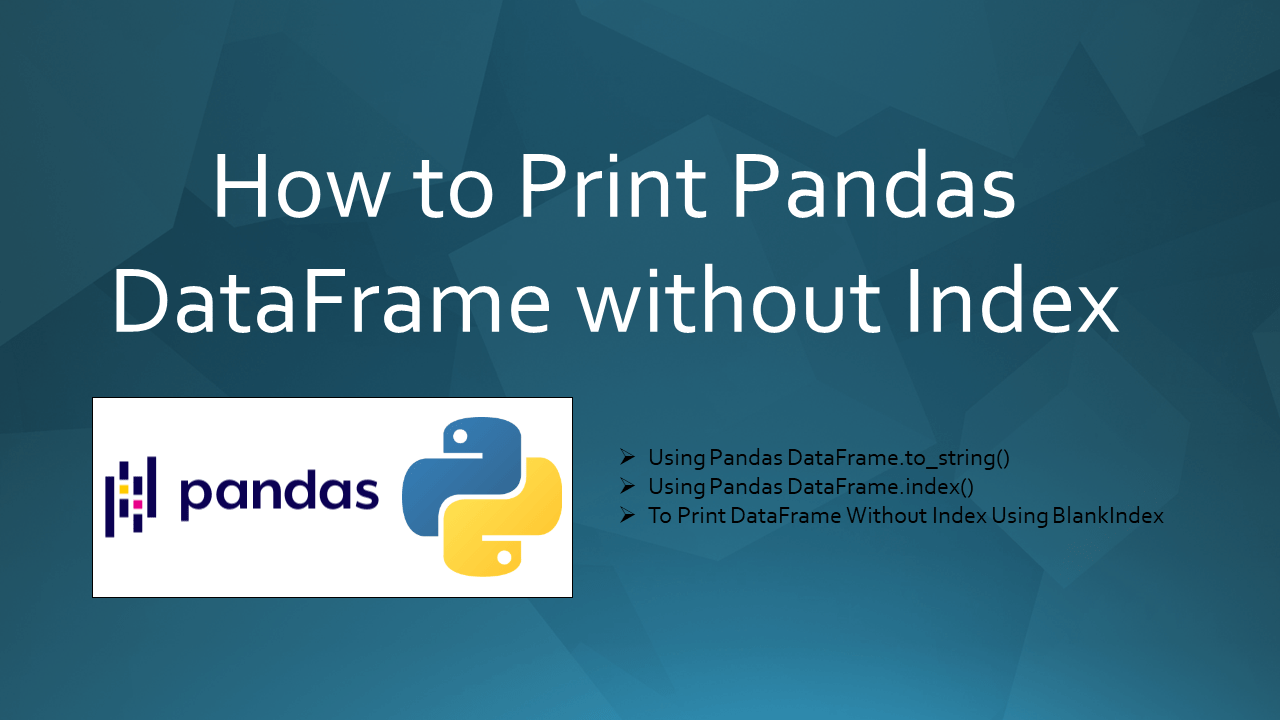# How to Print Pandas DataFrame without Index

To print the DataFrame without indices uses `DataFrame.to_string()` with `index=False` parameter. A pandas DataFrame has row indices/index and column names, when printing the DataFrame the row index is printed as the first column. In this article, I will explain how to print pandas DataFrame without index with examples.

## 1. Quick Examples of Print Pandas DataFrame without Index

If you are in a hurry, below are some quick examples of how to print pandas DataFrame without index.

``````
# Below are quick example
# Using DataFrame.to_string() to print without index
df2 = df.to_string(index=False)

# Using BlankIndex to print DataFrame without index
blankIndex=[''] * len(df)
df.index=blankIndex

# Using hide_index()
df.style.hide_index()
``````

Now, let’s create a DataFrame with a few rows and columns, execute these examples and validate results. Our DataFrame contains column names `Courses`, `Fee`, `Duration`, and `Discount`.

``````
import pandas as pd
technologies = {
'Courses':["Spark","PySpark","Python","pandas"],
'Fee' :[20000,25000,22000,30000],
'Duration':['30days','40days','35days','50days'],
'Discount':[1000,2300,1200,2000]
}
index_labels=['r1','r2','r3','r4']
df = pd.DataFrame(technologies,index=index_labels)
print(df)
``````

Yields below output.

``````
Courses    Fee Duration  Discount
r1    Spark  20000   30days      1000
r2  PySpark  25000   40days      2300
r3   Python  22000   35days      1200
r4   pandas  30000   50days      2000
``````

## 2. Using DataFrame.to_string() to Print DataFrame without Index

You can use `DataFrame.to_string(index=False)` on the DataFrame object to print. To result `DataFrame.to_string()` function is a string of the DataFrame without indices. The column names are retained as the first row.

``````
# Using DataFrame.to_string() to print without index
df2 = df.to_string(index=False)
print(df2)
``````

Yields below output.

``````
Courses   Fee Duration  Discount
Spark 20000   30days      1000
PySpark 25000   40days      2300
Python 22000   35days      1200
pandas 30000   50days      2000
``````

## 3. To Print DataFrame Without Index By Making Index empty

You can set the index as empty for each row, you can do this by creating an array with the empty string (one for each row in the DataFrame). and assign this to the `DataFrame.inde`x property.

``````
# Print DataFrame without index
blankIndex=[''] * len(df)
df.index=blankIndex
print(df)
``````

## 4. Use hide_index()

By using `hide_index()` method, you can hide the Index of the DataFrame. This is one of the best approach if you are using Python 3.7 or the latest.

``````
#Using hide Index
df.style.hide_index()
``````

## 5. Print DataFrame without Index on Jupyter Notebook

These days many developers and data analysts use Jupyter notebook to run the panda, so to remove and print the DataFrame without index use `display()` method.

``````
#Display without index on Jupyter.
display(df.hide_index())
``````

## 6. Write to CSV without Index

In case if you wanted to write a pandas DataFrame to a CSV file without Index, use param `index=False` in `to_csv()` method.

``````
# Write CSV file by ignoring Index.
print(df.to_csv(index=False))
``````

If you wanted to select some columns and ignore the index column.

``````
print(df.to_csv(columns=['Fee', 'Duration'], sep='\t', index=False))
``````

## 7. With Python 2.7

With Python 2.7, print has a different syntax. Use the below to print DataFrame in Python 2.7 version.

``````
# With older Python version
print df.to_string(index=False)
``````

Yields same output as above.

## Complete Example For Print DataFrame without Index

``````
import pandas as pd
technologies = {
'Courses':["Spark","PySpark","Python","pandas"],
'Fee' :[20000,25000,22000,30000],
'Duration':['30days','40days','35days','50days'],
'Discount':[1000,2300,1200,2000]
}
index_labels=['r1','r2','r3','r4']
df = pd.DataFrame(technologies,index=index_labels)
print(df)

# Using DataFrame.to_string() to print without index
df2 = df.to_string(index=False)
print(df2)

# Using BlankIndex to print DataFrame without index
blankIndex=[''] * len(df)
df.index=blankIndex
print(df)
``````

### Conclusion

In this article, you have learned how to print pandas DataFrame without an index or ignore index using `to_string()`, `index()` and `hide_index()` functions with examples.

Happy Learning !!

## References